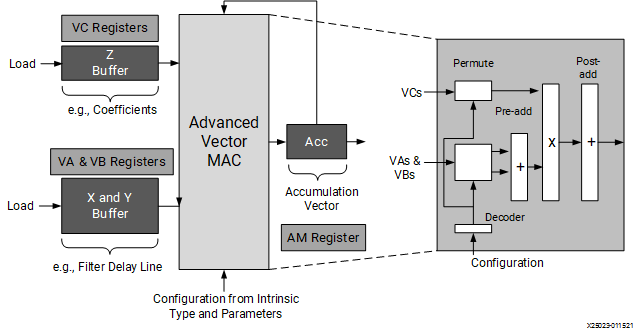# Vector Register Lane Permutations - 2022.1 English

## AI Engine Kernel Coding Best Practices Guide (UG1079)

Document ID
UG1079
Release Date
2022-05-25
Version
2022.1 English

The AI Engine fixed point vector units datapath consists of the following three separate and largely independently usable paths:

• Main MAC datapath
• Shift-round-saturate path
• Upshift path

The main multiplication path reads values from vector registers, permutes them in a user controllable fashion, performs optional pre-adding, multiplies them, and after some post-adding accumulates them to the previous value of the accumulator register.

While the main datapath stores to the accumulator, the shift-round-saturate path reads from the accumulator registers and stores to the vector registers or the data memory. In parallel to the main datapath runs the upshift path. It does not perform any multiplications but simply reads vectors, upshifts them and feeds the result into the accumulators. For details on the Fixed point and Floating point data paths refer to Versal ACAP AI Engine Architecture Manual (AM009). Details on the intrinsic functions that can be used to exercise these data paths can be found in the Versal ACAP AI Engine Intrinsics Documentation (UG1078).

As shown in the following figure, the basic functionality of MAC data path consists of vector multiply and accumulate operations between data from the X and Z buffers. Other parameters and options allow flexible data selection within the vectors and number of output lanes and optional features allow different input data sizes and pre-adding. There is an additional input buffer, the Y buffer, whose values can be pre-added with those from the X buffer before the multiplication occurs. The result from the intrinsic is added to an accumulator.

Figure 1. Functional Overview of the MAC Data PathThe operation can be described using lanes and columns. The number of lanes corresponds to the number of output values that will be generated from the intrinsic call. The number of columns is the number of multiplications that will be performed per output lane, with each of the multiplication results being added together. For example:

``````acc0 += z00*(x00+y00) + z01*(x01+y01) + z02*(x02+y02) + z03*(x03+y03)
acc1 += z10*(x10+y10) + z11*(x11+y11) + z12*(x12+y12) + z13*(x13+y13)
acc2 += z20*(x20+y20) + z21*(x21+y21) + z22*(x22+y22) + z23*(x23+y23)
acc3 += z30*(x30+y30) + z31*(x31+y31) + z32*(x32+y32) + z33*(x33+y33)
``````

In this case, four outputs are being generated, so there are four lanes and four columns for each of the outputs with pre-addition from the X and Y buffers.

The parameters of the intrinsics allow for flexible data selection from the different input buffers for each lane and column, all following the same pattern of parameters. The following section introduces the data selection (or data permute) schemes with detailed examples that include `shuffle` and `select` intrinsics. Details around the `mac` intrinsic and its variants are also discussed in the following sections.